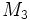# Category of differential manifolds with cobordisms

• The morphisms of the category are smooth cobordisms i.e. the morphisms from$M_1$ to$M_2$ are the cobordisms from$M_1$ to$M_2$
• Composition of morphisms is given as follows. Suppose$M_1 \sqcup M_2 \to \partial N$ is a smooth cobordism from$M_1$ to$M_2$, and$M_2 \sqcup M_3 \to \partial P$ is a smooth cobordism from$M_2$ to$M_3$. The cobordism from$M_1$ to$M_3$ is obtained by gluing$N$ and$P$ along the image of$M_2$, and not changing the maps from$M_1$ and$M_3$ to the respective parts.